Archive
Special IssuesVolume 1, Issue 1, May 2016, Page: 17-20
Conjugacy Class Lengths of Finite Groups with Prime Graph a Tree
Liguo He, Dept. of Math., Shenyang University of Technology, Shenyang, PR China
Yaping Liu, Dept. of Math., Shenyang University of Technology, Shenyang, PR China
Jianwei Lu, Dept. of Math., Shenyang University of Technology, Shenyang, PR China
Received: Apr. 11, 2016;       Accepted: May 3, 2016;       Published: May 28, 2016
Abstract
For a finite group G, we write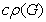to denote the prime divisor set of the various conjugacy class lengths of G and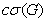the maximum number of distinct prime divisors of a single conjugacy class length of G. It is a famous open problem that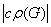can be bounded by. Let G be an almost simple group G such that the graph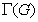built on element orders is a tree. By using Lucido’s classification theorem, we prove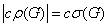except possibly when G is isomorphic to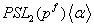, where p is an odd prime and α is a field automorphism of odd prime order f. In the exceptional case,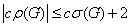. Combining with our known result, we also prove that for a finite group G witha forest, the inequality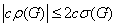is true.
Keywords
Prime Graph, Conjugacy Class Length, Almost Simple Group
Liguo He, Yaping Liu, Jianwei Lu, Conjugacy Class Lengths of Finite Groups with Prime Graph a Tree, Mathematics and Computer Science. Vol. 1, No. 1, 2016, pp. 17-20. doi: 10.11648/j.mcs.20160101.14
Reference

C. Casolo, Prime divisors of conjugacy class lengths in finite groups, Rend. Mat. Acc. Lincei, 1991, Ser. 9, 2: 111-113.

C. Casolo, Finite groups with small conjugacy classes, Manuscr. Math., 1994, 82: 171-189.

D. Chillig, M. Herzog, On the length of conjugacy classes of finite groups, J. Algebra, 1990, 131: 110-125.

L. Dornhoff, Group representation theory, Part A: Ordinary representation theory, Marcel Dekker, New York, 1971.

P. Ferguson, Connections between prime divisors of the conjugacy classes and prime divisors of, J. Algebra, 1991, 143: 25-28.

The GAP Group, GAP- Groups, algorithms, and programming, version 4.6,http://www.gap-system.org, 2013.

D. Gorenstein and R. Lyons, The local structure of finite groups of characteristic 2 type, Mem. Amer. Math. Soc. 276, 1983 (vol.42).

L. He, Y. Dong, Conjugacy class lengths of finite groups with disconnected prime graph, Int. J. Algebra, 2015, 9(5): 239 - 243.

B. Huppert, Character theory of finite groups, DeGruyter Expositions in Mathematics 25, Walter de Gruyter & Co.: Berlin. New York, 1998.

M. L. Lewis and D. L. White, Nonsolvable groups with no prime dividing three character degrees, J. Algebra, 2011, 336: 158-183.

M. S. Lucido, Groups in which the prime graph is a tree, Bollettino U.M.I., 2002, Ser. 8, 5-B: 131-148.

M. Suzuki, On a class of doubly transitive groups, Ann. Math., 1962, 75:105-145.

J. P. Zhang, On the lengths of conjugacy classes, Comm. Algebra, 1998, 26(8): 2395-2400.